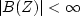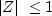Next: Mechanical interpretation Up: Z-plane, causality, and feedback Previous: Causality and the unit

# MINIMUM-PHASE FILTERS

Let bt denote a filter. Then at is its inverse filter if the convolution of at with bt is an impulse function. In terms of Z-transforms, an inverse is simply defined by A(Z) = 1/B(Z). Whether the filter A(Z) is causal depends on whether it is finite everywhere inside the unit circle, or really on whether B(Z) vanishes anywhere inside the circle. For example, B(Z)=1-2Z vanishes at Z = 1/2. There A(Z)=1/B(Z) must be infinite, that is to say, the series A(Z) must be nonconvergent at Z = 1/2. Thus, as we have just seen, at is noncausal. A most interesting case, called minimum phase," occurs when both a filter B(Z) and its inverse are causal. In summary,


causal:forcausal inverse:forminimum phase: 		 both above conditions

The reason the interesting words minimum phase'' are used is given in chapter.Next: Mechanical interpretation Up: Z-plane, causality, and feedback Previous: Causality and the unit
Stanford Exploration Project
10/21/1998